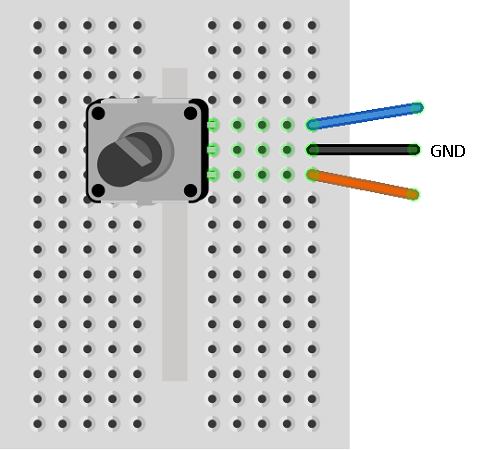# BBC micro:bit Rotary Encoder Class

## Introduction

A rotary encoder has a knob to rotate a little bit like a rotary potentiometer. One big difference is that the knob can be rotated continuously. When I first connected a rotary encoder to the micro:bit, I used the button pins (5 and 11) because they had been configured differently to the other GPIO pins and in a way that was useful for rotary encoders. Since then, additional features have been added to MicroPython making it easy to use them on other pins.

This page contains a class for working with rotary encoders. I wrote this to help work with the circuit shown below, which has two rotary encoders.## Connecting A Rotary Encoder

There are 3 pins for a rotary encoder, usually referred to as A, B and GND. Connect them up to your choice of GPIO pins as follows.## Rotary Encoder Class

```from microbit import *

class rotary:
def __init__(self,pina,pinb):
self.pina = pina
self.pinb = pinb
pina.set_pull(pina.PULL_UP)
pinb.set_pull(pinb.PULL_UP)
self.lastA = False
# returns -1 (ccw), 0 no change, 1 (cw)
check = self.lastA
self.lastA = a
if not a and check:
if b:
return -1
else:
return 1
else:
return 0
```

## Using The Class

In the image at the top of the page, the rotary encoder on the left is connected to pins 0 and 1. The one on the right is connected to pins 8 and 12. The program allows the user to move a dot around the LED matrix by rotating the left encoder to move horizontally and the right one to move vertically.

```from microbit import *

class rotary:
def __init__(self,pina,pinb):
self.pina = pina
self.pinb = pinb
pina.set_pull(pina.PULL_UP)
pinb.set_pull(pinb.PULL_UP)
self.lastA = False
# returns -1 (ccw), 0 no change, 1 (cw)
check = self.lastA
self.lastA = a
if not a and check:
if b:
return -1
else:
return 1
else:
return 0

lft = rotary(pin0,pin1)
rgt = rotary(pin8,pin12)
x = 2
y = 2
display.set_pixel(x,y,9)
while True:
if l!=0 or r!=0:
display.set_pixel(x,y,0)
if l==1:
x += 1
elif l==-1:
x -= 1
if r==1:
y += 1
elif r==-1:
y -= 1
x = max(0, min(x, 4))
y = max(0, min(y, 4))
display.set_pixel(x,y,9)
sleep(5)
```